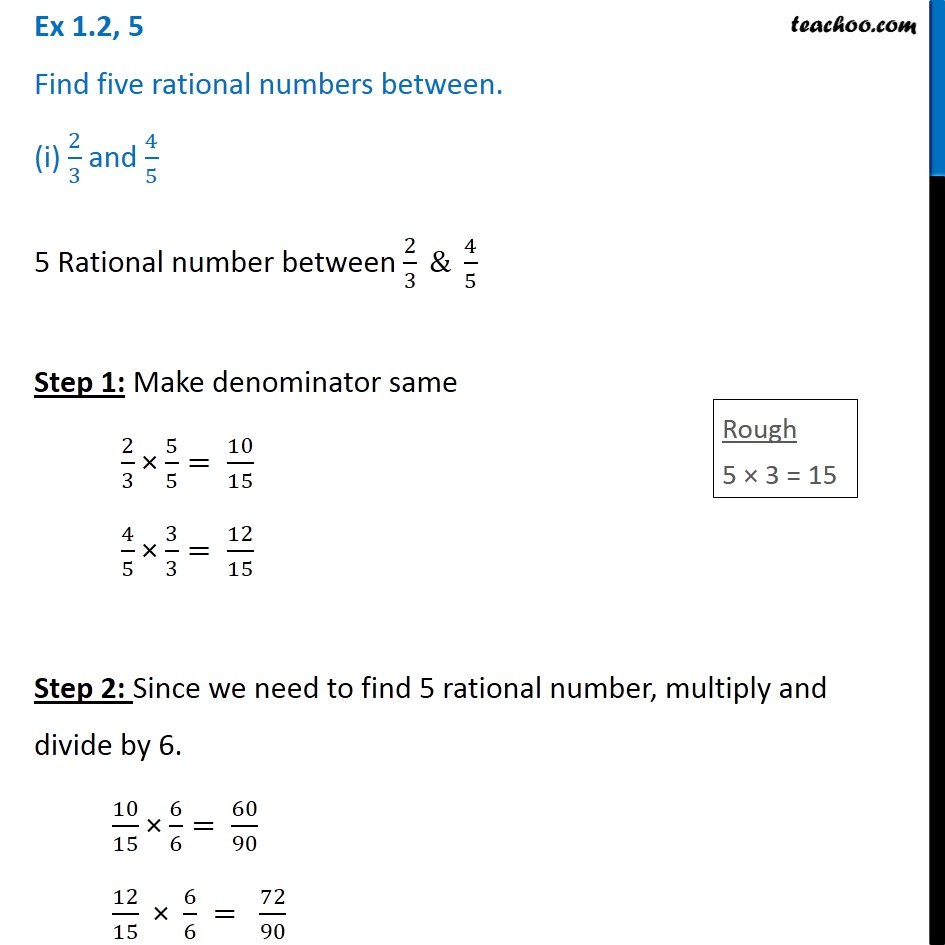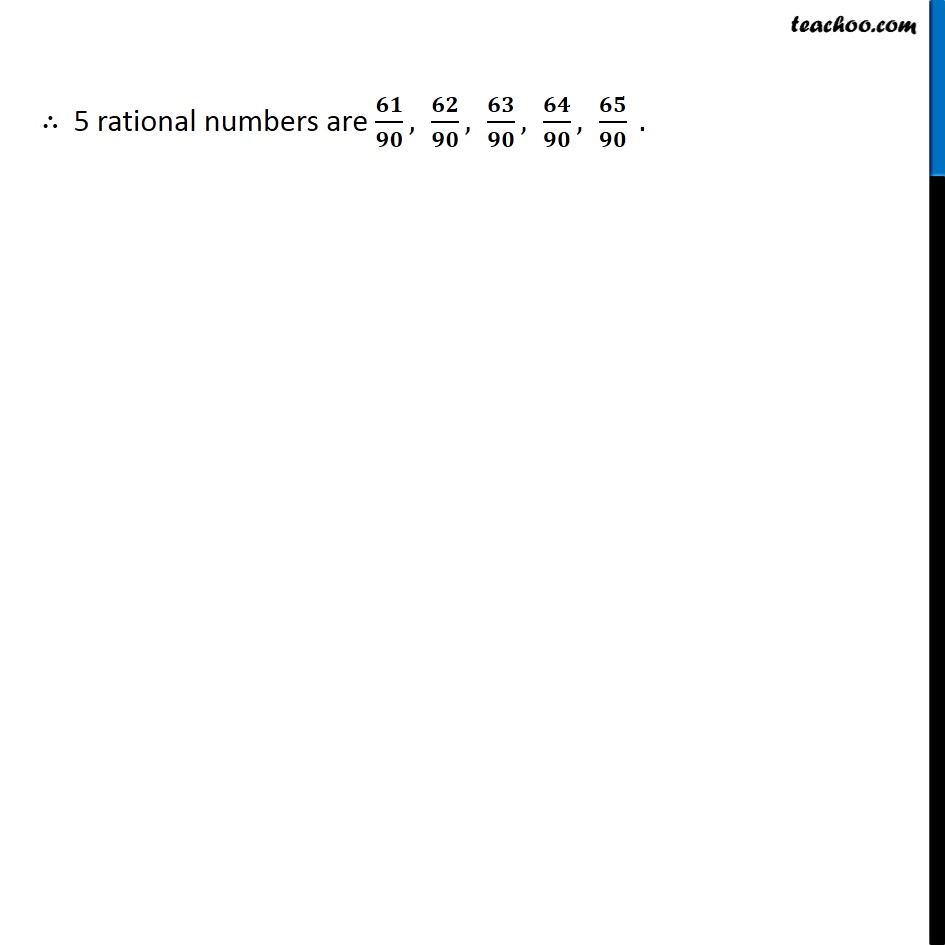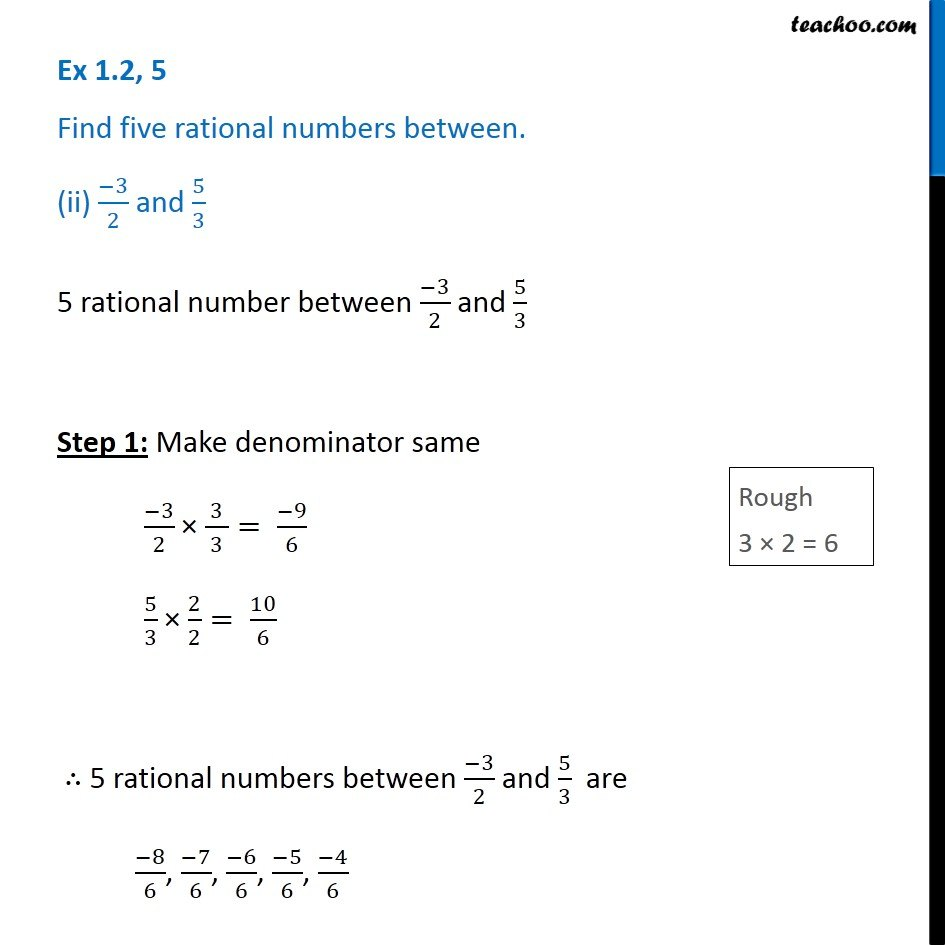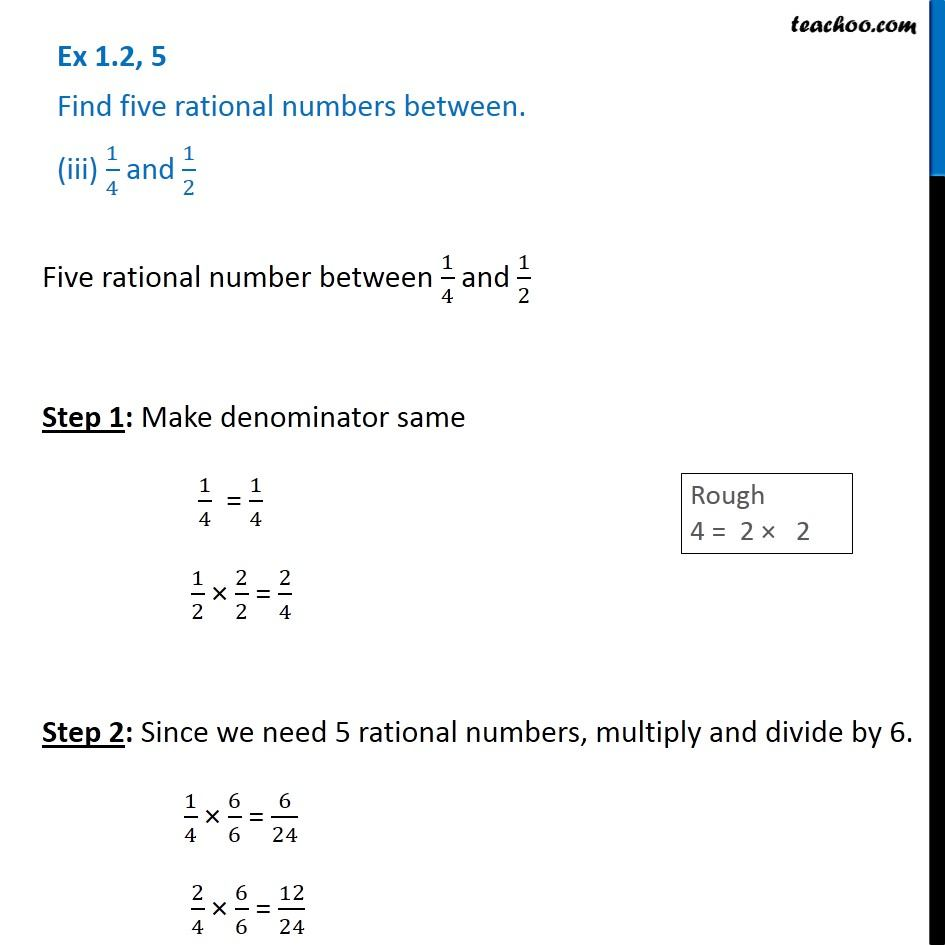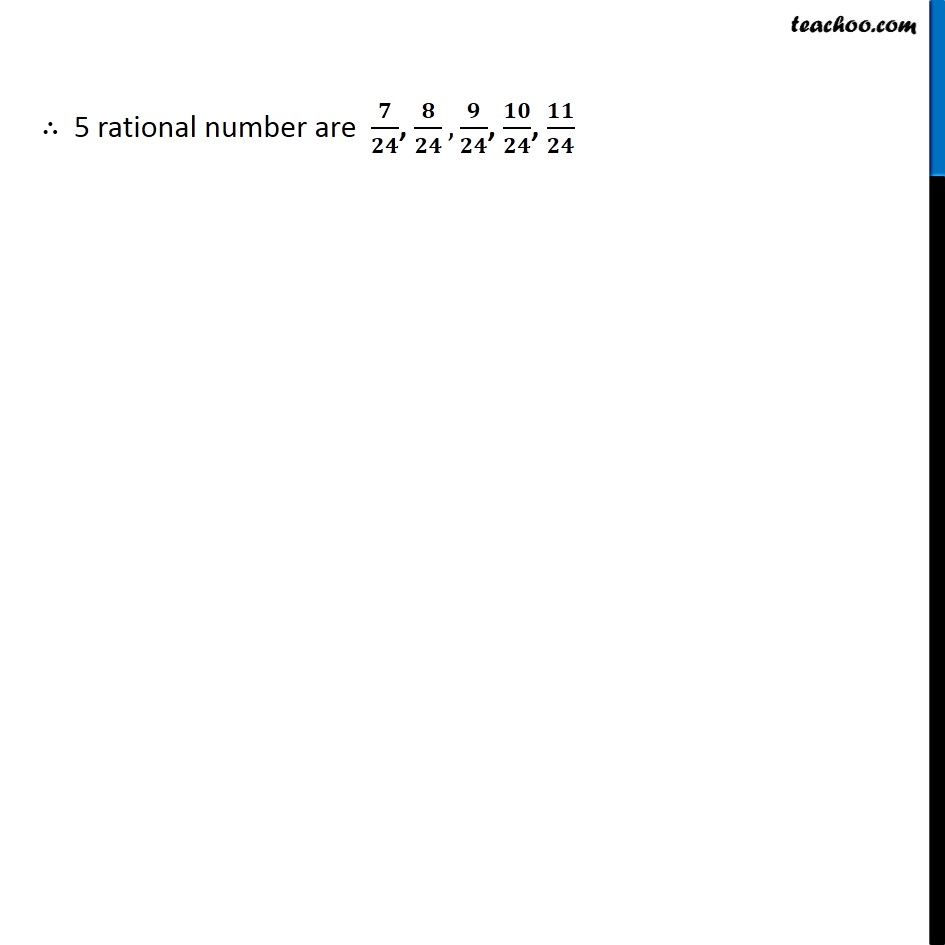1. Chapter 1 Class 8 Rational Numbers
2. Serial order wise
3. Ex 1.2

Transcript

Ex 1.2, 5 Find five rational numbers between. (i) 2/3 and 4/5 5 Rational number between 2/3 & 4/5 Step 1: Make denominator same 2/3 × 5/5= 10/15 4/5 × 3/3= 12/15 Step 2: Since we need to find 5 rational number, multiply and divide by 6. 10/15 × 6/6= 60/90 12/15 × 6/6 = 72/90 ∴ 5 rational numbers are 𝟔𝟏/𝟗𝟎, 𝟔𝟐/𝟗𝟎, 𝟔𝟑/𝟗𝟎, 𝟔𝟒/𝟗𝟎, 𝟔𝟓/𝟗𝟎 . Ex 1.2, 5 Find five rational numbers between. (ii) (−3)/2 and 5/3 5 rational number between (−3)/2 and 5/3 Step 1: Make denominator same (−3)/2 × ( 3 )/3= (−9)/6 5/3 × 2/2= 10/6 ∴ 5 rational numbers between (−3)/2 and 5/3 are (−8)/6, (−7)/6, (−6)/6, (−5)/6, (−4)/6 Ex 1.2, 5 Find five rational numbers between. (iii) 1/4 and 1/2 Five rational number between 1/4 and 1/2 Step 1: Make denominator same 1/4 = 1/4 1/2 × 2/2 = 2/4 Step 2: Since we need 5 rational numbers, multiply and divide by 6. 1/4 × 6/6 = 6/24 2/4 × 6/6 = 12/24 ∴ 5 rational number are 𝟕/𝟐𝟒, 𝟖/𝟐𝟒,𝟗/𝟐𝟒, 𝟏𝟎/𝟐𝟒, 𝟏𝟏/𝟐𝟒

Ex 1.2